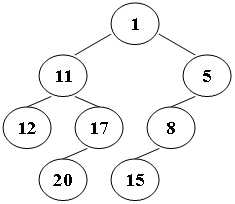Suppose that all the keys in a binary tree are distinct positive integers. A unique binary tree can be determined by a given pair of postorder and inorder traversal sequences. And it is a simple standard routine to print the numbers in level-order. However, if you think the problem is too simple, then you are too naive. This time you are supposed to print the numbers in "zigzagging order" -- that is, starting from the root, print the numbers level-by-level, alternating between left to right and right to left. For example, for the following tree you must output: 1 11 5 8 17 12 20 15.## Input Specification:

Each input file contains one test case. For each case, the first line gives a positive integer N (≤30), the total number of nodes in the binary tree. The second line gives the inorder sequence and the third line gives the postorder sequence. All the numbers in a line are separated by a space.

## Output Specification:

For each test case, print the zigzagging sequence of the tree in a line. All the numbers in a line must be separated by exactly one space, and there must be no extra space at the end of the line.

## Sample Input:

8
12 11 20 17 1 15 8 5
12 20 17 11 15 8 5 1

## Sample Output:

1 11 5 8 17 12 20 15

## 解答

#include<iostream>
#include<vector>
#include<queue>
using namespace std;

vector<int> in, post;

struct node {
int key, depth;
node *lchild, *rchild;
};

node *createTree(int inl, int inr, int postl, int postr) {
if (inl > inr) return NULL;
int i;
node* root = new node();
root->key = post[postr];
for (i = inl; i <= inr; i++)
if (in[i] == root->key) break;
int leftNum = i - inl;
root->lchild = createTree(inl, i - 1, postl, postl + leftNum - 1);
root->rchild = createTree(i + 1, inr, postl + leftNum, postr - 1);
return root;
}
void bfs(node* root) {
queue<node*> q;
vector<vector<node*>> v(1);
q.push(root);
int currentCnt = 1, nextCnt = 0, i = 0, depth = 0;
while (!q.empty()) {
node* tmp = q.front();
v[depth].push_back(tmp);
q.pop();
i++;
if (tmp->lchild) {
q.push(tmp->lchild);
nextCnt++;
}
if (tmp->rchild) {
q.push(tmp->rchild);
nextCnt++;
}
if (i == currentCnt) {
depth++;
currentCnt = nextCnt, i = 0, nextCnt = 0;
v.resize(v.size() + 1);
}
}
printf("%d", v->key);
for (int i = 1; i < v.size(); i++) {
if (i % 2)
for (auto it = v[i].begin(); it != v[i].end(); it++)
printf(" %d", (*it)->key);
else
for (auto it = v[i].rbegin(); it != v[i].rend(); it++)
printf(" %d", (*it)->key);
}
}

int main()
{
int n;
cin >> n;
in.resize(n), post.resize(n);
for (int i = 0; i < n; i++) cin >> in[i];
for (int i = 0; i < n; i++) cin >> post[i];
node *tree = createTree(0, n - 1, 0, n - 1);
bfs(tree);
return 0;
}